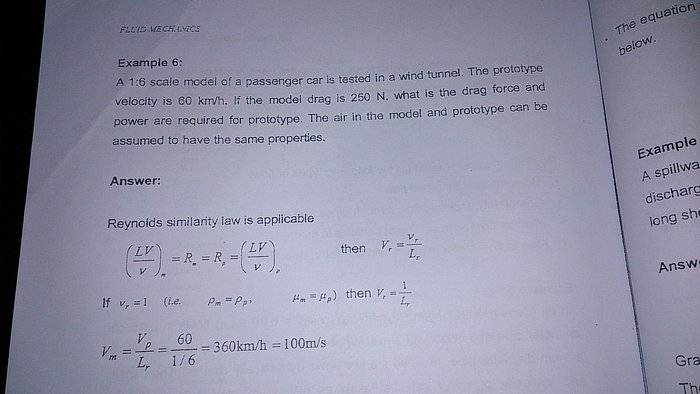# Geometric similarity

## Homework Statement

in this question , Lm / Lp = 1/ 6 ? or Lp / Lm = 1/6 ? Lp = length of prototype , Lm = Length of model## The Attempt at a Solution

i really have no idea... can someone help please?

phinds
Gold Member
2021 Award
If you have no idea at all then the question arises, how do you come to be working on a problem that you have no idea how to answer? What level of school are you in? Where is the problem from? We don't spoon-feed answers here, we try to help you solve a problem yourself, but we need more background to even be able to give you a hint that would be meaningful to you.

•welovephysics
If you have no idea at all then the question arises, how do you come to be working on a problem that you have no idea how to answer? What level of school are you in? Where is the problem from? We don't spoon-feed answers here, we try to help you solve a problem yourself, but we need more background to even be able to give you a hint that would be meaningful to you.
i am in uni now , and the notes is from my book , IMO , it should be Lp / Lm = 1/6 , am i right ?
can you help ?

i dont think the working provided is correct
IMO , it should be (Vr)( Lr )= (nu)r

nu = kinematic viscosity
since nu is constant , so ,

Vp / Vm = Lm / Lp
60 / Vm = 1/6

so , Vm = 360km/h , am i right ?

thus , force has formula of ρ(L^3)(velocity) , so force is directly proportional to v ,

Fp / Fm = Vp / Vm
Fp / 250 = 60/360 , Fp = 41.7N , is it correct ?